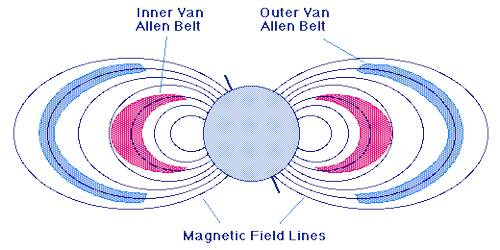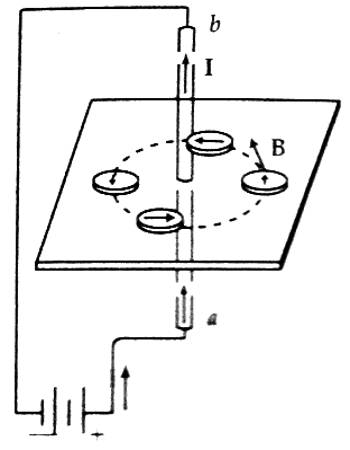# Explanation of Magnetic Field

Explanation of Magnetic field

Hans Christian Oersted was a Danish scientist who explored the relationship between electric current and magnetism. Current is the flow of electrons and is how we hardness electricity. Currents create their own magnetic fields in closed loops, which magnets are known to induce or create current, in wires. In Oersted’s experiment, it is seen that magnetic needles deflection if they are kept near a current-carrying wire. Deflection is more near the wire and less at far point and no deflection is seen at a long distance.

Explanation: Suppose current ‘I’ is flowing through a wire ab. Due to this flow of current magnetic field around the wire has been produced. Now, if a magnetic needle is placed around the wire at different positions of the same distance from the wire, the needle will give equal deflection: but the directions of deflection will be different [Figure]. If the points of equal deflection of the needle are connected by a curve, it will be a circle in two dimensions [In fig. it is shown by dot-dot lines]. Centering the wire if curves at different distances are drawn along equal deflection points, then innumerable circles can be drawn. These circular curves are closed curves – they neither start nor stop in the middle. These lines or curves are called magnetic field lines or magnetic induction lines (simply induction line.). The tangent drawn at any point of the line gives the direction of the magnetic field at that point. So magnetic field lines can be defined in the following way.Definition: Magnetic field lines are some dosed curved lines drawn on magnetic field and tangents are drawn on them at any point gives the direction of magnetic field at that point.